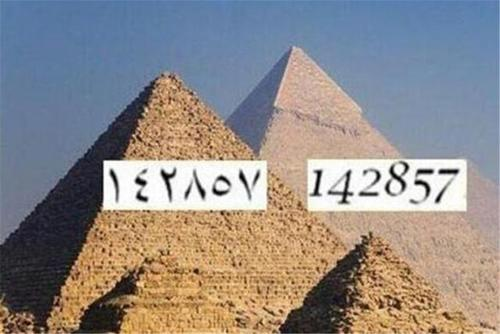# 埃及金字塔未解之谜数字

1、神奇的数字

142857 × 1 = 14285(7) 1*7=7

142857 × 2 = 28571(4) 2*7=14

142857 × 3 = 42857(1) 3*7=21

142857 × 4 = 57142(8) 4*7=28

142857 × 5 = 71428(5) 5*7=35

142857 × 6 = 85714(2) 6*7=42

142857 × 7 = 99999(9) 7*7=49

142857 × 8 = 114285(6) 8*7=56

142857 × 9 = 128571(3) 9*7=63

142857 × 10 = 142857(0) 10*7=70

1-6的结果，横竖都有(142857)没有0369 神奇吧。有点像“独数”不过是没有0369的独数。

20408122449的前五位+上后六位的得数是多少呢?

20408 + 122449 = 142857

..............